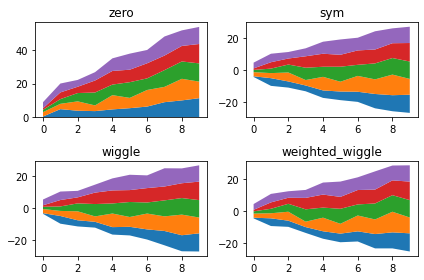The `stackplot()` function of matplotlib allows to make stacked area chart. It provides a baseline argument that allows to custom the position of the areas around the baseline. Four possibilities are exist, and they are represented here. This chart is strongly inspired from the Hooked answer on this stack overflow question, thanks to him!

``````# libraries
import numpy as np
import matplotlib.pyplot as plt
import seaborn as sns

# Create data
X = np.arange(0, 10, 1)
Y = X + 5 * np.random.random((5, X.size))

# There are 4 types of baseline we can use:
baseline = ["zero", "sym", "wiggle", "weighted_wiggle"]

# Let's make 4 plots, 1 for each baseline
for n, v in enumerate(baseline):
if n<3 :
plt.tick_params(labelbottom='off')
plt.subplot(2 ,2, n + 1)
plt.stackplot(X, *Y, baseline=v)
plt.title(v)
plt.tight_layout()``````## Contact & Edit

👋 This document is a work by Yan Holtz. You can contribute on github, send me a feedback on twitter or subscribe to the newsletter to know when new examples are published! 🔥